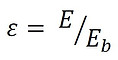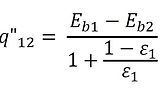###### Workshop #13 - Radiation IntroductionThe emissivity ε of a surface is a measure of the radiation heat flux or emissive power E that is emitted relative to the maximum from a “black body”. This black body emissive power is defined from basic physics as

where the value of the constant σ = 5.67 × 10^-8 W/m^2-K when the temperature is specified in Kelvin degrees. The emissivity is then simply

When there are multiple surfaces exchanging radiation, all of the surfaces are both receiving and emitting energy. If the two surfaces are both black (ε = 1) and very near to each other, the radiation heat flux from surface 1 to surface 2 is simply

If one of the surfaces is gray (ε < 1), an additional thermal resistance needs to be included as

This reduces to

Remember this is the radiation flux for two surfaces very close together with one surface black and one gray.

You are asked to measure the emissivity of a metallic surface. To do this, you are provided with a heated plate that is painted black on one-half of the plate. The other half is polished metal. Note that the plate is at a uniform temperature because it is thick and has high thermal conductivity. It should be about 90°C to easily feel the radiation. Compare the heat transfer from the metal (gray) surface to that of the painted (black) surface (ε = 1) at the same conditions. Use the heat flux sensor to measure the radiation emission from the plate. Mount the sensor onto your small aluminum block to provide a heat sink. Use a piece of black (electrical) tape to give a nearly black surface (ε = 1) to the heat flux sensor.

1. Put your hand close to the plate first over one half and then the other to feel the difference between the black and gray surfaces. Note how it feels if you move farther away. DO NOT TOUCH the plate. It is VERY hot.

2. Without touching the plate, put your free thermocouple between the plate and heater to measure the plate temperature Hold your heat flux sensor close, but not touching the plate. Take about 20 seconds of data for each of the black and gray surfaces and save in one file. Record the temperatures of the plate and sensor and the measured heat flux from each half of the plate to the sensor below. Find the corresponding emissivity of the polished metal.

3. How does the gray surface feel different than the black surface at the same temperature? Why is this?

4. To simplify the system analysis neglect convection and assume that the surfaces are very close (view factor between the sensor and plate is unity). Based on the equations in the introduction for this case the ratio of the heat flux for the two cases should be directly proportional to the ratio of the emissivities of the surfaces. Because the sensor is assumed black (ε = 1), this should give the gray surface emissivity as equal to the ratio of the gray surface heat flux to the black surface heat flux.

Evaluate, εgray

5. Explain why the plate did not feel as hot as you moved your hand away from the hot surface. (How does the view factor change?)

6. Based on the measured temperatures, find the black body emissive powers of the two surfaces, Eb = σT^4. From the equations in the Introduction, calculate the predicted sensor heat flux from the black surface to the sensor, qʺblack. Explain any differences between the calculated and measured values of heat flux.###### Workshop #13 - Radiation Results